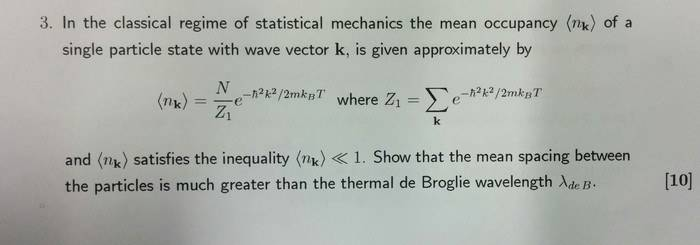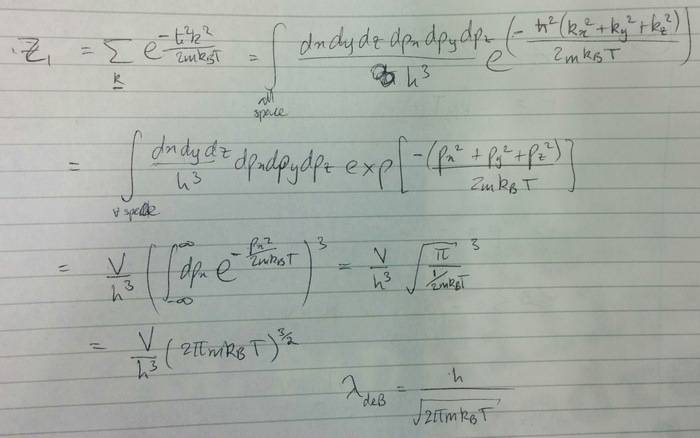# Show interparticle spacing is much greater than wavelength

I have a past paper question from statistical physics:By assuming that ##\hbar^2 k^2=p^2##, I arrived at the result:
The interparticle spacing, ##a^3 =\frac{V}{N}## is
$$a^3 >> e^{-\frac{p^2}{2mk_B T}} \lambda_{deB}^3$$

Is my assumption correct? and does the result complete the purpose of the question?